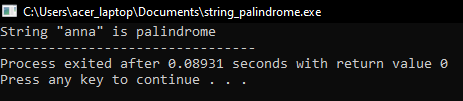# How to Compare String and Checking Palindrome in C

## How to Compare String and Checking Palindrome in C Language

In this article, I am going to discuss How to Compare String and Checking Palindrome in C Language with Examples. Please read our previous article where we discussed How to Reverse a String in C Language with Examples.

##### How to Compare String and Checking Palindrome in C Language

In this article, we will see how to compare strings and check whether a string is a palindrome or not. We’ll explain to you what does it mean my palindrome. And also explain to you how to find whether a string is a palindrome or not. First, let us look at how to compare two strings.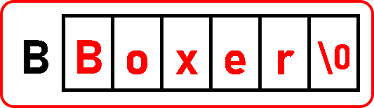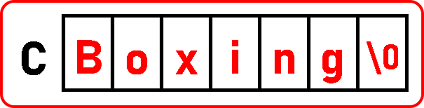We have taken two strings “Boxer” and “Boxing”. So, if we look at these strings, ‘Box’ is common in both the strings. After ‘x’ they are different. We need a method to know whether two strings are the same or different.

Let’s see how to compare them. We should scan one letter at a time from both the strings. So, we will take ‘i’ and ‘j’ as character pointers to scan every letter in both the strings: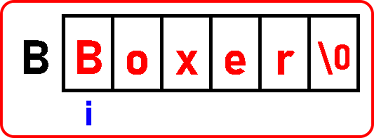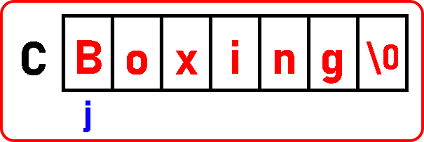‘i’ and ‘j’ are pointing at on letter ‘B’. Both are pointing to the same letter. Increment ‘i’ and ‘j’. Again, they are pointing to the same letter ‘o’. Increment them again. Now they are pointing letter ‘x’. Both the above arrays have different letters after the letter ‘x’.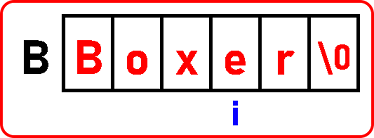Now, ‘i’ and ‘j’ are pointing to different letters. Now, they are not matching. So, no need to continue further. If any alphabet at corresponding locations is not matching then those two strings are not the same. So, the answer is the above two strings are not equal. We can also check which string comes first and which comes next. The smaller thing comes first in the dictionary. Greater string comes next.

So, if we check which comes first. In the above two strings, ‘Box’ is common to both the string, and after this, there is ‘e’ and ‘i’ letters. Now we have to check which letter’s ASCII code comes first. So here e comes first then i. So ‘e’ is smaller than ‘i’.

So, in Dictionary ‘Boxing’ will come first and then ‘Boxer’. We can say that ‘Boxing’ a string is smaller than this ‘Boxer’. So here we can compare two strings and the result is that ‘Box’ is common to both the string and the ‘Boxing’ is smaller than ‘Boxer’. This was the example where the strings were not matching.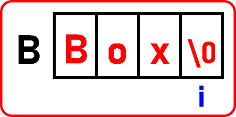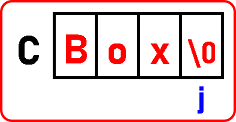Now we have taken two strings of the same set of characters. This is the case where strings are matching. We should stop the over procedure if we have reached ‘\0’.

So, the procedure will go on comparing the alphabets one by one, and when it will stop? There are two situations if a mismatch is found then it will stop or if any one of the strings is ending it will stop. Below is the code to perform this procedure:

##### Program for Comparing String in C Language:
#include <stdio.h>
#include <stdlib.h>
int main ()
{
char B[] = “Boxer”;
char C[] = “Boxing”;
int i, j;
printf (“1st String is \”%s\”\n”, B);
printf (“2nd String is \”%s\”\n”, C);
for (i = 0, j = 0; B[i] != ‘\0’ && C[j] != ‘\0’; i++, j++)
{
if (B[i] != C[j])
break;
}
if (B[i] == C[j])
printf (“Both strings are equal\n”);
else if (B[i] < C[j])
printf (“1st string is smaller\n”);
else
printf (“1st string is greater\n”);
return 0;
}

For inside the loop what we should do? We should check if strings are matching or not, if they’re matching, we don’t have to do anything if they are not matching then we should stop the procedure. After coming out of the loop, we should see whether they are equal.

###### Output: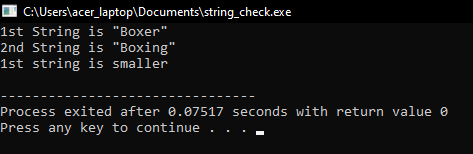##### How to check whether a string is a palindrome or not?

Now next we will show you what is palindrome and how to check whether a string is a palindrome or not. Let us know what is a palindrome or string said to be a palindrome. If you reverse a string and it remains the same then it is known as a palindrome.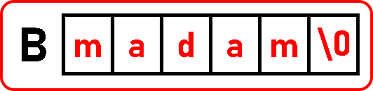For example, I have a string here that is madam. When we reverse this string, again it has formed the same string “madam”. If you reverse a string and if it remains the same then it is a palindrome. Like “naman”, “neven”, “anna” is examples of palindrome string.

Our problem is if a string is given then we have to find out whether it’s a palindrome or not. Now the procedure is: First of all, we want a reverse copy of that string. So, if we have a string in an array then we have to reverse copy it into another array. Then after we have to compare two strings: the original one and the reverse copied string. So, if they are equal then it is a palindrome. Let’s implement this in our code:

##### Program to Check whether a string is a palindrome or not in C language:
#include <stdio.h>
#include <stdlib.h>
int main ()
{
char B[] = “anna”;
char t;
bool palindrome = true;
int i, j;
printf (“String \”%s\” is “, B);
for (j = 0; B[j] != ‘\0’; j++)
{
// no code here
}
j = j – 1;
for (i = 0; i < j; i++, j–)
{
if (B[i] != B[j])
{
palindrome = false;
}
}
if (palindrome)
printf (“palindrome”);
else
printf (“not palindrome”);
return 0;
}

Output: